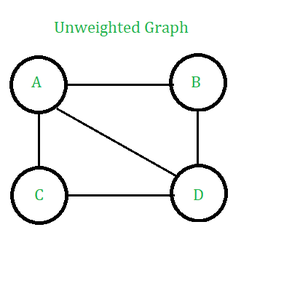GeeksforGeeks App
Open AppBrowser
Continue

## Related Articles

An unweighted graph is a graph in which the edges do not have weights or costs associated with them. Instead, they simply represent the presence of a connection between two vertices.

In this article we will explore the simplicity of unweighted graphs, where connections between vertices are represented without the complexity of edge weights. From social networks to web pages, unweighted graphs have a wide range of applications in various fields. Discover the advantages and disadvantages of this type of graph and how it can be used to solve problems.Unweighted Graph

Applications of Unweighted Graph:

• Unweighted graphs are used to represent data that are not related in terms of magnitude.
• Unweighted graphs are used to represent computation flow.
• Representation of image segmentation, where pixels are represented as nodes and edges represent adjacency relationships.
• Representation of state spaces in decision-making processes and problem-solving in AI.
• Representation of information networks, such as the World Wide Web.

Real-Time Applications of Unweighted Graph:

• Unweighted graphs can be used to solve puzzles.
• It can be used to represent a circuit diagram.
• It can be used in social media sites to find whether two users are connected or not.
• It is used in Hamiltonian graphs which have many practical applications like genome mapping to combine many tiny fragments of genetic code.
• Used in computer networks as it represents the connections between computers in a network as an unweighted graph.
• Used in social networks as it representing the relationships between individuals in a social network as an unweighted graph.
• It represents the possible moves in a game as an unweighted graph.

• Unweighted graphs can be used to implement tree data structures.
• Unweighted graphs are used in many algorithms like DFS and BFS.
• Helps in optimal visualization of interrelated problems which are not related in terms of magnitude.
• Unweighted graphs are simpler to work with than weighted graphs, as they do not require the storage or manipulation of edge weights.
• Unweighted graphs are suitable for problems where the edge weights are not relevant or not known.
•  Unweighted graphs are simple and easy to understand as they only consider the presence or absence of a relationship between two nodes.
• Unweighted graphs are space efficient, as they only require a small amount of memory to store the information about the presence of an edge.
• Unweighted graphs are easy to interpret, as they only represent the presence of a relationship and do not require interpretation of edge weights.